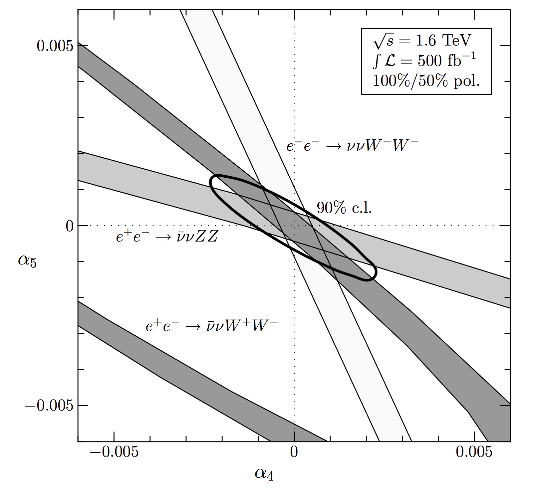May 12, 2006

Mo’ Better Bounds

One of the novelties (for me, at least), in writing a paper in a well-worn field like precision electroweak measurements, is that a lot of people have a prior stake in the subject. Our recent paper got a flood of very positive comments.

All very gratifying. But, at the end of last week, came one comment which made us realize that our bounds (from forward-scattering dispersion relations) could be considerably strengthened. So we spent most of the intervening week redoing our analysis, and rewriting our paper to incorporate the new, stronger bounds.

Forward scattering produced the bounds \array{\arrayopts{\colalign{right left}} \bar \ell_1+2 \bar \ell_2&\geq \frac{1}{32} (-36+9 \pi )\\ \bar \ell_2&\geq \frac{1}{48} (-92+39 \pi )\\ \bar \ell_1+3 \bar \ell_2&\geq 0.911166 } Theoretically, this was notable because the shape of the allowed region differed from that predicted by the classical superluminality analysis, which can also be thought of as modified classical propagation resulting from (tree-level) forward scattering off a nontrivial background field.

The new, stronger bounds are \array{\arrayopts{\colalign{right left}} \bar \ell_1+2 \bar \ell_2&\geq 157/40\\ \bar \ell_2&\geq 27/20\\ \bar \ell_1+3 \bar \ell_2 &\geq 211/40. } Unlike the previous case, the 3rd bound is redundant to the other two. A comparison of the old and new bounds can be seen in the figure below.

The region allowed by the dispersion relation analysis. Forward scatter dispersion relations exclude the crimson and burnt orange regions. The new bounds exclude the light blue region as well.

Theoretically, you might not find the new bounds all that interesting an improvement over the old ones. But, when you compare them with what might be measured experimentally at the ILC, the new, tighter bounds become somewhat more interesting experimentally.

There’s more work to be done, but one can kinda get a rough idea by comparing our bounds with the following graph from Boos et al. To make the comparison, $\alpha_4 \sim \overline{\ell}_2/192\pi^2$ $\alpha_5 \sim \overline{\ell}_1/384\pi^2$.1-$\sigma$ exclusion contours for all three processes in the $\alpha_4/\alpha_5$ plane, based on the hypothesis $\alpha_4=\alpha_5=0$. All cuts have been applied and detection efficiencies are included. The closed contour curve is the 90% exclusion limit obtained by combining the $e^+e^-\to \bar\nu\nu W^+W^-$ and $e^+e^-\to \bar\nu\nu ZZ$ channels. (from Boos et al)

What one would like to show, for instance, is that a measurement which yielded that 90% CL ellipse implies a violation of our bounds …

Posted by distler at May 12, 2006 11:36 PM

TrackBack URL for this Entry:   https://golem.ph.utexas.edu/cgi-bin/MT-3.0/dxy-tb.fcgi/808